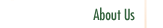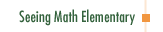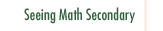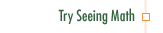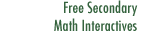Pre-Algebra: Pan Balance Equations

In Pre-Algebra: Pan Balance Equations, a fifth grade class manipulates concrete representations of equivalence, using pan balances, to understand the nature of equations and operations on equations. Students investigate a model for solving equations using one and two variables.

Participants in this course:

• Examine the nature of algebraic equivalence.
• Understand the multiple meanings of the equals sign.
• See how using diagrams to solve problems helps students understand algebraic symbols.
• Examine questioning strategies that support concept clarification, reflection, and discussion.
• Explore recent research into how students learn to reason algebraically.
• Examine how diagrams can build student understanding of equivalence and traditional algebraic symbols.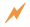Click to Get Seeing Math™ ElementaryCourse Listing Effective Questioning in the Mathematics Classroom Foundations of EffectiveMathematics Teaching Formative Assessment in the Mathematics Classroom Number & Operations: Division with Remainders Number & Operations: The Magnitude of Fractions Number & Operations: Broken Calculator Geometry: 2D and 3D Figures Geometry: Calculating Area of a Triangle Data Analysis and Probability: Using Data to Make Predictions Data Analysis and Probability: Measures of Center Pre-Algebra: Pan Balance Equations Pre-Algebra: Patterns and Functions